Math & Science Home | Proficiency Tests | Mathematical Thinking in Physics | Aeronauts 2000

 CONTENTS Introduction Fermi's Piano Tuner Problem How Old is Old? If the Terrestrial Poles were to Melt... Sunlight Exerts Pressure Falling Eastward What if an Asteroid Hit the Earth Using a Jeep to Estimate the Energy in Gasoline How do Police Radars really work? How "Fast" is the Speed of Light? How Long is a Light Year? How Big is a Trillion? "Seeing" the Earth, Moon, and Sun to Scale Of Stars and Drops of Water If I Were to Build a Model of the Cosmos... A Number Trick Designing a High Altitude Balloon Pressure in the Vicinity of a Lunar Astronaut Space Suit due to Outgassing of Coolant Water Calendar Calculations Telling Time by the Stars - Sidereal Time Fields, an Heuristic Approach The Irrationality ofThe Irrationality ofThe Number (i)i Estimating the Temperature of a Flat Plate in Low Earth Orbit Proving that (p)1/n is Irrational when p is a Prime and n>1 The Transcendentality ofIdeal Gases under Constant Volume, Constant Pressure, Constant Temperature and Adiabatic Conditions Maxwell's Equations: The Vector and Scalar Potentials A Possible Scalar Term Describing Energy Density in the Gravitational Field A Proposed Relativistic, Thermodynamic Four-Vector Motivational Argument for the Expression-eix=cosx+isinx Another Motivational Argument for the Expression-eix=cosx+isinx Calculating the Energy from Sunlight over a 12 hour period Calculating the Energy from Sunlight over actual full day Perfect Numbers-A Case Study Gravitation Inside a Uniform Hollow Sphere Further note on Gravitation Inside a Uniform Hollow Sphere Pythagorean Triples Black Holes and Point Set Topology Additional Notes on Black Holes and Point Set Topology Field Equations and Equations of Motion (General Relativity) The observer in modern physics A Note on the Centrifugal and Coriolis Accelerations as Pseudo Accelerations - PDF File On Expansion of the Universe - PDF File

#### Motivational Argument for the Expression: eix = cos x + i sin x

Problem: Show that eix = cos x + i sin x, where i =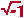Solution: Let us turn to the theory of differential equations. The equation

y'' + y = 0

(where the prime notation symbolizes differentiation with respect to x) has a solution of the form y =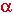cos x +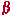sin x. This same differential equation also has a solution of the form y =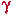eix +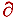e-ix. [This latter form is obtained by assuming the ansatz, y = em, and using it in the original equation to obtain the condition on m that m2 + 1 = 0, from which m = ± i.]

We may now equatecos x +sin x =eix +e-ix (eq. 1)

We wish to solve for eix. Differentiating once and multiplying the result by -i gives

(- i) [(-sin x) + (cos x)] =eix -e-ix
or
i [sin x -cos x] =eix -e-ix (eq.2)

Adding eqs. 1 and 2 gives

2eix= (- i) cos x + (+ i) sin x (eq. 3)
or
eix = (* - i*) cos x + (* + i*) sin x (eq. 3a)

where* =/2and* =/2. We must now determine values for* and*. We do so by setting x = 0 and observing that ei0 = e0 = 1. Also, cos 0 = 1 and sin 0 = 0. In this case, eq. 3a reduces to

1 =* - i*

Equating the real and imaginary parts immediately gives* = 1, and* = 0.

With these values, eq. 3a yields the required expression

eix = cos x + i sin x Q.E.D.